Back to the main Index

# Post-processing tools for electron band energies¶

In this notebook we discuss how to plot electron band structures and density of states (DOS) using the netcdf files produced by Abinit.

For the tutorial, we will use the netcdf files shipped with AbiPy. The function abidata.ref_file returns the absolute path of the reference file. In your scripts, you have to replace data.ref_file("abipy_filename") with a string giving the location of your netcdf file.

Alteratively, one can use the abiopen.py script to open the file inside the shell with the syntax:

abiopen.py out_GSR.nc



This command will start the ipython interpreter so that one can interact directly with the GsrFile object (named abifile inside ipython). To generate a jupyter notebook use:

abiopen.py out_GSR.nc -nb



For a quick visualization of the data, use the --expose options:

abiopen.py out_GSR.nc -e



In :
# Use this at the beginning of your script so that your code will be compatible with python3
from __future__ import division, print_function, unicode_literals

import warnings
warnings.filterwarnings("ignore")  # Ignore warnings

from abipy import abilab
abilab.enable_notebook() # This line tells AbiPy we are running inside a notebook
# Import abipy reference data.
import abipy.data as abidata

# This line configures matplotlib to show figures embedded in the notebook.
# Replace inline with notebook in classic notebook
%matplotlib inline

# Option available in jupyterlab. See https://github.com/matplotlib/jupyter-matplotlib
#%matplotlib widget


## The GSR File¶

The GSR file (mnemonics: Ground-State Results) is a netcdf file with the results produced by SCF or NSCF ground-state calculations (band energies, forces, energies, stress tensor). To open a GSR file, use the abiopen function defined in abilab:

In :
gsr = abilab.abiopen(abidata.ref_file("si_scf_GSR.nc"))


The gsr object has a Structure:

In :
print(gsr.structure)

Full Formula (Si2)
Reduced Formula: Si
abc   :   3.866975   3.866975   3.866975
angles:  60.000000  60.000000  60.000000
Sites (2)
#  SP       a     b     c  cartesian_forces
---  ----  ----  ----  ----  -----------------------------------------------------------
0  Si    0     0     0     [-5.89948302e-27 -1.93366148e-27  2.91016902e-27] eV ang^-1
1  Si    0.25  0.25  0.25  [ 5.89948302e-27  1.93366148e-27 -2.91016902e-27] eV ang^-1

Abinit Spacegroup: spgid: 227, num_spatial_symmetries: 48, has_timerev: True, symmorphic: True


and an ElectronBands object with the band energies, the occupation factors, the list of k-points:

In :
print(gsr.ebands)

================================= Structure =================================
Full Formula (Si2)
Reduced Formula: Si
abc   :   3.866975   3.866975   3.866975
angles:  60.000000  60.000000  60.000000
Sites (2)
#  SP       a     b     c  cartesian_forces
---  ----  ----  ----  ----  -----------------------------------------------------------
0  Si    0     0     0     [-5.89948302e-27 -1.93366148e-27  2.91016902e-27] eV ang^-1
1  Si    0.25  0.25  0.25  [ 5.89948302e-27  1.93366148e-27 -2.91016902e-27] eV ang^-1

Abinit Spacegroup: spgid: 227, num_spatial_symmetries: 48, has_timerev: True, symmorphic: True

Number of electrons: 8.0, Fermi level: 5.598 (eV)
nsppol: 1, nkpt: 29, mband: 8, nspinor: 1, nspden: 1
smearing scheme: none, tsmear_eV: 0.272, occopt: 1
Direct gap:
Energy: 2.532 (eV)
Initial state: spin=0, kpt=[+0.000, +0.000, +0.000], weight: 0.002, band=3, eig=5.598, occ=2.000
Final state:   spin=0, kpt=[+0.000, +0.000, +0.000], weight: 0.002, band=4, eig=8.130, occ=0.000
Fundamental gap:
Energy: 0.562 (eV)
Initial state: spin=0, kpt=[+0.000, +0.000, +0.000], weight: 0.002, band=3, eig=5.598, occ=2.000
Final state:   spin=0, kpt=[+0.375, +0.375, +0.000], weight: 0.012, band=4, eig=6.161, occ=0.000
Bandwidth: 11.856 (eV)
Valence maximum located at:
spin=0, kpt=[+0.000, +0.000, +0.000], weight: 0.002, band=3, eig=5.598, occ=2.000
Conduction minimum located at:
spin=0, kpt=[+0.375, +0.375, +0.000], weight: 0.012, band=4, eig=6.161, occ=0.000



A GSR file produced by a self-consistent run, contains the values of the total energy, the forces, and the stress tensor at the end of the SCF cycle:

In :
print("energy:", gsr.energy, "pressure:", gsr.pressure)

energy: -241.23646831544198 eV pressure: -5.211617575719521 GPa


To get a summary of the most important results:

In :
print(gsr)

================================= File Info =================================
Name: si_scf_GSR.nc
Directory: /Users/gmatteo/git_repos/abipy/abipy/data/refs/si_ebands
Size: 14.83 kb
Access Time: Sat Oct 12 16:49:15 2019
Modification Time: Wed Mar 20 16:53:35 2019
Change Time: Wed Mar 20 16:53:35 2019

================================= Structure =================================
Full Formula (Si2)
Reduced Formula: Si
abc   :   3.866975   3.866975   3.866975
angles:  60.000000  60.000000  60.000000
Sites (2)
#  SP       a     b     c  cartesian_forces
---  ----  ----  ----  ----  -----------------------------------------------------------
0  Si    0     0     0     [-5.89948302e-27 -1.93366148e-27  2.91016902e-27] eV ang^-1
1  Si    0.25  0.25  0.25  [ 5.89948302e-27  1.93366148e-27 -2.91016902e-27] eV ang^-1

Abinit Spacegroup: spgid: 227, num_spatial_symmetries: 48, has_timerev: True, symmorphic: True

Stress tensor (Cartesian coordinates in GPa):
[[5.21161758e+00 7.86452261e-11 0.00000000e+00]
[7.86452261e-11 5.21161758e+00 0.00000000e+00]
[0.00000000e+00 0.00000000e+00 5.21161758e+00]]

Pressure: -5.212 (GPa)
Energy: -241.23646832 (eV)

============================== Electronic Bands ==============================
Number of electrons: 8.0, Fermi level: 5.598 (eV)
nsppol: 1, nkpt: 29, mband: 8, nspinor: 1, nspden: 1
smearing scheme: none, tsmear_eV: 0.272, occopt: 1
Direct gap:
Energy: 2.532 (eV)
Initial state: spin=0, kpt=[+0.000, +0.000, +0.000], weight: 0.002, band=3, eig=5.598, occ=2.000
Final state:   spin=0, kpt=[+0.000, +0.000, +0.000], weight: 0.002, band=4, eig=8.130, occ=0.000
Fundamental gap:
Energy: 0.562 (eV)
Initial state: spin=0, kpt=[+0.000, +0.000, +0.000], weight: 0.002, band=3, eig=5.598, occ=2.000
Final state:   spin=0, kpt=[+0.375, +0.375, +0.000], weight: 0.012, band=4, eig=6.161, occ=0.000
Bandwidth: 11.856 (eV)
Valence maximum located at:
spin=0, kpt=[+0.000, +0.000, +0.000], weight: 0.002, band=3, eig=5.598, occ=2.000
Conduction minimum located at:
spin=0, kpt=[+0.375, +0.375, +0.000], weight: 0.012, band=4, eig=6.161, occ=0.000



The different contributions to the total energy are stored in a dictionary:

In :
print(gsr.energy_terms)

Term                  Value
e_localpsp            -68.62575616682041 eV
e_eigenvalues         4.453251781809304 eV
e_ewald               -226.94266538122858 eV
e_hartree             14.989583868349118 eV
e_corepsp             2.262480246606245 eV
e_corepspdc           0.0 eV
e_kinetic             80.6603511758275 eV
e_nonlocalpsp         52.15353045099117 eV
e_entropy             0.0 eV
entropy               0.0 eV
e_xc                  -95.73399250916707 eV
e_xcdc                0.0 eV
e_paw                 0.0 eV
e_pawdc               0.0 eV
e_elecfield           0.0 eV
e_magfield            0.0 eV
e_fermie              5.5984532787385985 eV
e_sicdc               0.0 eV
e_exactX              0.0 eV
h0                    0.0 eV
e_electronpositron    0.0 eV
edc_electronpositron  0.0 eV
e0_electronpositron   0.0 eV
e_monopole            0.0 eV


At this point, we don't need this file anymore so we close it with:

In :
gsr.close()


## Plotting band structures¶

Let's open the GSR file produced by a NSCF calculation done on a high-symmetry k-path and extract the electronic band structure.

A warning is issued by pymatgen about the structure not being standard. Be aware that this might possibly affect the automatic labelling of the boundary k-points on the k-path. So, check carefully the k-point labels on the figures that are produced in such case. In the present case, the labelling is correct.

In :
with abilab.abiopen(abidata.ref_file("si_nscf_GSR.nc")) as nscf_gsr:
ebands_kpath = nscf_gsr.ebands


Now we can plot the band energies with:

In :
# The labels for the k-points are found in an internal database.
ebands_kpath.plot(with_gaps=True, title="Silicon band structure");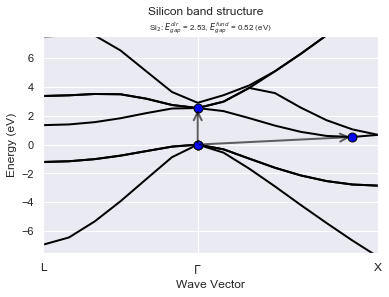Alternatively you can use the optional argument klabels to define the mapping reduced_coordinates --> name of the k-point and pass it to the plot method

In :
klabels = {
(0.5, 0.0, 0.0): "L",
(0.0, 0.0, 0.0): "$\Gamma$",
(0.0, 0.5, 0.5): "X"
}

# ebands_kpath.plot(title="User-defined k-labels", band_range=(0, 5), klabels=klabels);


Let's have a look at our k-points by calling kpoints.plot()

In :
ebands_kpath.kpoints.plot();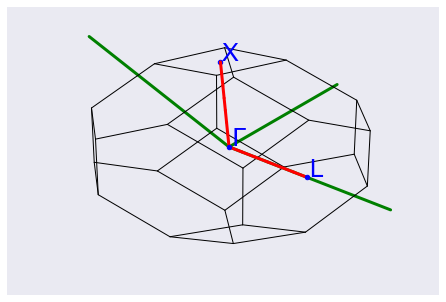and the crystalline structure with:

In :
ebands_kpath.structure.plot();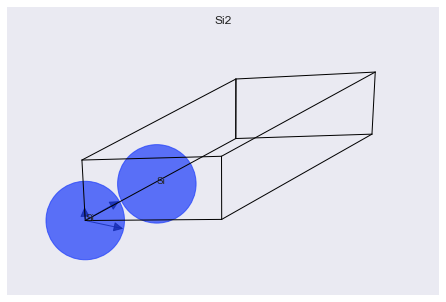The same piece of code works if you replace the GSR.nc file with e.g. a WFK.nc file in netcdf format (actually any netcdf file with an ebands object). The main advantage of the GSR file is that it is lightweight (no wavefunctions).

## DOS with the Gaussian technique¶

Let's use the eigenvalues and the k-point weights stored in gs_ebands to compute the DOS with the Gaussian method. The method is called without arguments so we use default values for the broadening and the step of the linear mesh.

In :
 with abilab.abiopen(abidata.ref_file("si_scf_GSR.nc")) as scf_gsr:
ebands_kmesh = scf_gsr.ebands

edos = ebands_kmesh.get_edos()
print(edos)

nsppol: 1, nelect: 8.0
Fermi energy: 5.839906241274078 (eV) (recomputed from nelect):

In :
edos.plot();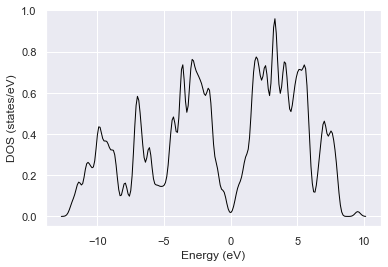In :
print("[ebands_kmesh] is_ibz:", ebands_kmesh.kpoints.is_ibz, "is_kpath:", ebands_kmesh.kpoints.is_path)
print("[ebands_kpath] is_ibz:", ebands_kpath.kpoints.is_ibz, "is_kpath:", ebands_kpath.kpoints.is_path)

[ebands_kmesh] is_ibz: True is_kpath: False
[ebands_kpath] is_ibz: False is_kpath: True


To plot bands and DOS on the same figure:

In :
ebands_kpath.plot_with_edos(edos, with_gaps=True);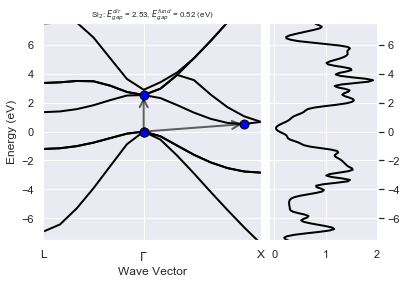To plot the DOS and the integrated DOS (IDOS), use:

In :
edos.plot_dos_idos();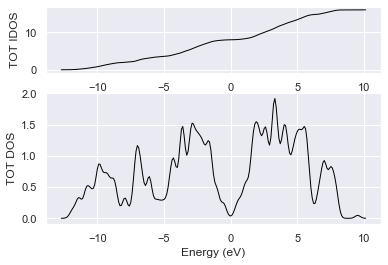The gaussian broadening can significantly change the overall shape of the DOS. If accurate values are needed (e.g. the DOS at the Fermi level in metals), one should perform an accurate convergence study with respect to the k-point mesh. Here we show how compute the DOS with different values of the gaussian smearing for fixed k-point sampling and plot the results:

In :
# Compute the DOS with the Gaussian method and different values of the broadening
widths = [0.1, 0.2, 0.3, 0.4]

edos_plotter = ebands_kmesh.compare_gauss_edos(widths, step=0.1)


To plot the results on the same figure, use:

In :
edos_plotter.combiplot(title="e-DOS as function of the Gaussian broadening");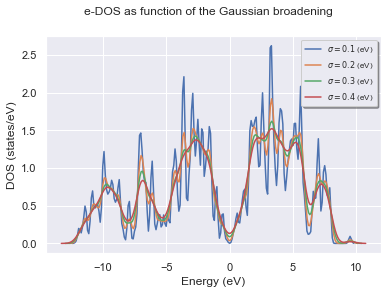while gridplot generates a grid of subplots:

In :
edos_plotter.gridplot();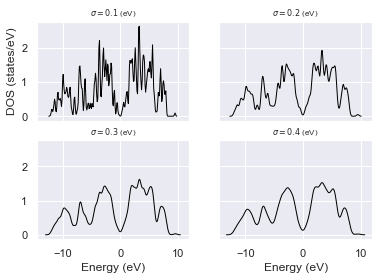## Joint density of states¶

This example shows how to plot the different contributions to the electronic joint density of states of Silicon. Select the valence and conduction bands to be included in the JDOS. Here we include valence bands from 0 to 3 and the first conduction band (4).

In :
vrange = range(0,4)
crange = range(4,5)

# Plot data
ebands_kmesh.plot_ejdosvc(vrange, crange);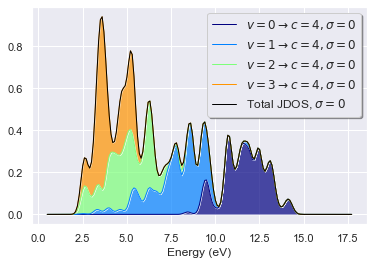In :
ebands_kpath.plot_transitions(omega_ev=3.0);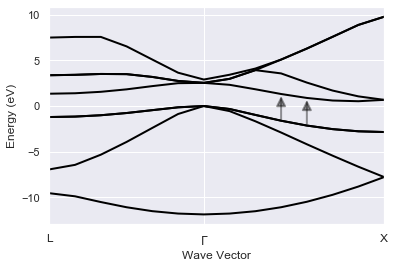In :
ebands_kmesh.plot_transitions(omega_ev=3.0);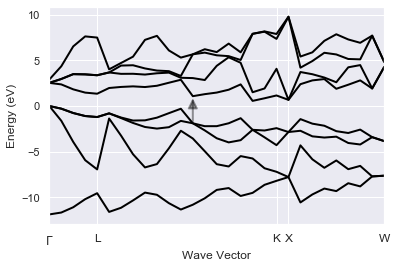## Plotting the Fermi surface¶

In :
with abilab.abiopen(abidata.ref_file("mgb2_kmesh181818_FATBANDS.nc")) as fbnc_kmesh:
mgb2_ebands = fbnc_kmesh.ebands

# Build ebands in full BZ.
mgb2_eb3d = mgb2_ebands.get_ebands3d()


There are three bands crossing the Fermi level of $MgB_2$ (band 2, 3, 4):

In :
mgb2_ebands.boxplot();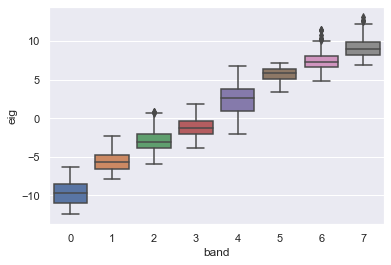Let's use matplotlib to plot the isosurfaces corresponding to the Fermi level (default):

In :
# Warning: requires skimage package, rendering could be slow.
mgb2_eb3d.plot_isosurfaces();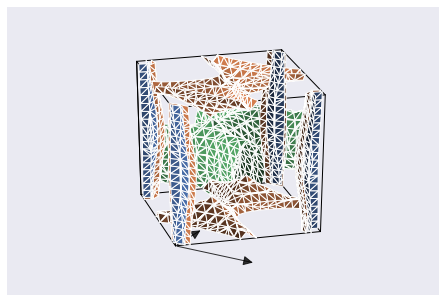In :
#ebands_kpath.plot_scatter3d(band=3);
#ebands_kmesh.plot_scatter3d(band=3);


## Analyzing multiple GSR files with robots¶

TODO

Back to the main Index